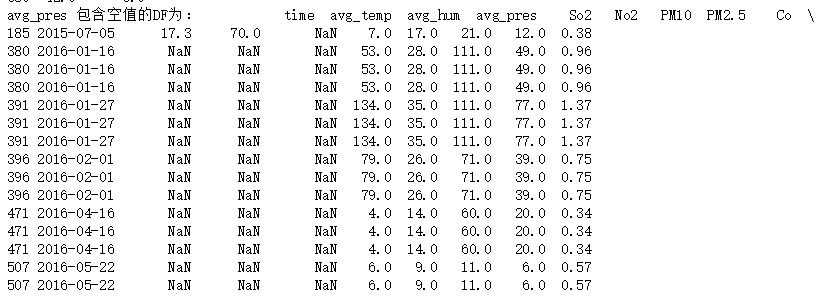• 判断数据中是否空值 统计空值/非空值数量 根据空值筛选数据 查找空值索引 删除空值 dropna()函数 填充空值fillna()函数 为了便于描述，定义本文示例数据为如下结构： df = pd.DataFrame([[1, np.nan], [np...
本文整理了数据中空值的处理操作，主要内容如下：
判断数据中是否有空值
统计空值/非空值数量
根据空值筛选数据
查找空值索引
删除空值 dropna()函数
填充空值fillna()函数

为了便于描述，定义本文示例数据为如下结构：
df = pd.DataFrame([[1, np.nan], [np.nan, 4], [5,6],[np.nan,7]],columns=["A","B"])
df  #定义示例数据df


判断数据中是否有空值
pandas isnull()函数
df.isnull()    #返回df中各元素是否为空的同df大小的数据框

df["A"].isnull()  #判断A列中空值情况

df[["A","B"]].isnull()  # 指定多列进行空值判断，对于本文实例，下述代码效果同df.isnull() 
pandas notnull()函数
df.notnull()    #判断df中各元素是否 不是 空值

df["A"].isnull()  #判断A列中非空值情况

df[["A","B"]].isnull()  # 指定多列进行非空值判断，对于本文实例，下述代码效果同df.notnull() 
numpy np.isnan() 函数
np.isnan(df)   # 等同于df.isnull()

np.isnan(df["A"])   # 等同于 df["A"].isnull()

np.isnan(df[["A","B"]])  # 等同于 df[["A","B"]].isnull()

统计空值/非空值数量
df.isnull().sum()  # 统计每列的空值数量

df.notnull().sum()  # 统计每列的非空值数量

df["A"].count()     # A列 非空数量
df.count()         # 统计所有列的非空值数量
df.count(axis=1)   # 每行非空值数量，axis=1

df["A"].sum()      # A列 元素数值之和
根据空值筛选数据
# 筛选出A列为空的所有行
df[df.A.isnull()]
df[df["A"].isnull()]

# 筛选出A列非空的所有行
df[df.A.notnull()]
df[df["A"].notnull()]

# 筛选出df中存在空值的行
df[df.isnull().values==True] 
查找空值索引
np.where(np.isnan(df))   # df中空值所在的行索引及列索引

np.where(np.isnan(df.A))    # df中A列空值所在的行索引

删除空值 dropna()函数

df.dropna()   # 删除存在空值的行，默认axis=0按行，how=any每行存在一个空值就执行删除行操作

df.dropna(axis=1)  # 删除存在空值的列

df.dropna(how="all")  # 删除所有列都为空值的特定行

df.dropna(how = "any")   # 删除存在空值的行

# 对特定列空值进行删除

df.dropna(how="any",subset=["A"])  # 删除A列中存在空值的行

df.dropna(how="any",subset=["A","B"])  # 删除A,B列中只要有一列存在空值的行

#将删除操作作用于原数据，修改替换原数据

df.dropna(how="all",subset=["A","B"],inplace=True)  # 删除A,B列都为空值的行,并替换原数据

填充空值fillna()函数
# 用指定的数字来填充
df.fillna(0)   # 用0来填充df中的空值

# 用指定的函数统计值来填充
df.fillna(df.mean())  # 用df中数据的平均值来填充空值

df.fillna(df.mean()["A"])   #指定用A列数据均值来填充df中空值

df.fillna(df.sum())   # 用df中数据的和来填充空值

# 用字典来填充
values = {'A': 0, 'B': 1}   # A列空值用0填充，B列空值用1填充
df.fillna(value=values)

# 用指定字符串来填充空值
df.fillna("unkown")

# 不同的填充方式{‘backfill’, ‘bfill’, ‘pad’, ‘ffill’, None}
# 每列的空值，用其列下方非空数值填充
df.fillna(method="backfill")
df.fillna(method="bfill")   # 同backfill
# 每列的空值，用其所在列上方非空数值填充，若上方没有元素，保持空值
df.fillna(method="ffill")

#limit参数设置填充空值的最大个数
df.fillna(0,limit=1)  # 每列最多填充1个空值，超过范围的空值依然为空

#inplace参数空值是否修改原数据df
df.fillna(0,inplace=True)  # inplace为true，将修改作用于原数据



展开全文python pandas
• 通过numpy生成空值，并通过代码快速解决空值问题。
标题 利用numpy处理空值数据
当我们用Python处理数据时，经常会出现NaN值，此时可以用过简单的赋值有效处理空值问题。

明确需求：处理数据文件中的空值。 分析逻辑： 1.遍历数据中的所有列，判断是否有空值 2.如果有空值就把数据列中非空值取出来，求平均数 3.把非空平均数赋值给空值

首先导入numpy，并创建一组数据。
import numpy as np
from numpy import NaN
data=np.arange(24,dtype='float').reshape(4,6)

把其中的一行数字替换成NaN值。
data[2,1:]=NaN
print(data)
[[ 0.  1.  2.  3.  4.  5.]
[ 6.  7.  8.  9. 10. 11.]
[12. nan nan nan nan nan]
[18. 19. 20. 21. 22. 23.]]

遍历数据。
for x in range(data.shape):
data_col=data[:,x]
nan_num=np.count_nonzero(data_col!=data_col)

判断空值，并把非空平均数赋值给NaN值。
	if nan_num!=0:
data_not_nan_num=data_col[data_col==data_col]
data_col[np.isnan(data_col)]=np.mean(data_not_nan_num)
print(data)
[[ 0.  1.  2.  3.  4.  5.]
[ 6.  7.  8.  9. 10. 11.]
[12.  9. 10. 11. 12. 13.]
[18. 19. 20. 21. 22. 23.]]

此时空值就无了。除了用平均值，还可以使用任何我们想替换的数据来赋值。
展开全文大数据 python 数据分析
• 关于np.nan需要注意的地方总结如下： ...来判断。只能用np.isnan()来操作。 np.nan的数据类型是float。 import numpy as np np.nan == np.nan Out: False aa = np.array([1,2,3,np.nan,np.nan,4,5,np.n...
关于np.nan需要注意的地方总结如下：
np.nan不是空对象。对列表中的nan进行操作时不能用"==np.nan"来判断。只能用np.isnan()来操作。np.nan的数据类型是float。
import numpy as np

np.nan == np.nan
Out: False

aa = np.array([1,2,3,np.nan,np.nan,4,5,np.nan])
aa
Out: array([  1.,   2.,   3.,  nan,  nan,   4.,   5.,  nan])

aa[aa==np.nan] = 100  #错误方式
aa
Out: array([  1.,   2.,   3.,  nan,  nan,   4.,   5.,  nan])

aa[np.isnan(aa)] = 100  #对nan操作的正确方式
aa
Out: array([   1.,    2.,    3.,  100.,  100.,    4.,    5.,  100.])

type(np.nan)
Out: float


展开全文Python nan
• 最近发现，对于pandas中的NaN值是无法直接使用 ==np.nan来判断空值，以为在数学中，inf==inf，而nan != nan，可以通过编写代码中发现下面的情况。 &gt;&gt;&gt; np.nan == np.nan False 那么我们在...
最近发现，对于pandas中的NaN值是无法直接使用 ==np.nan来判断空值，以为在数学中，inf==inf，而nan != nan，可以通过编写代码中发现下面的情况。
>>> np.nan == np.nan
False

那么我们在判断一个值为空值的可以下面的形式
>>print(np.isnan(np.nan))
True

可以得知，对空值的判断不能使用直接的数学比较形式==，而需要使用numpy自带的方法进行判断。
比如获取某列有缺失的行信息，可以使用以下代码,可以打印有空值的行
print(i,'包含空值的DF为：',the_have_null_df[np.isnan(the_have_null_df[col_name])])展开全文• 最近在做数据处理的时候，遇到个让我欲仙欲死的问题，那就是数据中的空值该如何获取。 我的目的本来是获取数据中的所有非零且非空值，然后再计算获得到的所有数据计算均值，再用均值把0和空值填上。这个操作让我...pandas Python
• numpy中的nan并不是空对象，其实际上是numpy.float64对象，所以我们不能误认为其是空对象，从而用bool(np.nan)去判断是否空值，这是不对的。 对于pandas中的空值，我们该如何判断，并且有哪些我们容易掉进去的...
• 本文主要介绍用numpy和pandas检查ndarray中是否存在缺失值的方法以及运行时间(缺失值的表示方式为np.nan) import numpy as np import pandas as pd # 随机初始化一个(100, 100)的数组 data = np.random.rand(100, ...python 数据分析
• 对于numpy数组判断是否为空，可以用数组中元素个数size是否为0 : if lat_value.size: print(lat_value.size) #1 另外，对于loc取出的DataFrame，取出其中的Values得到一个多维数组，: lat=sim_poi.loc...
• isnan = np.isnan(x) # 判断每个元素是不是nan,返回[False,False,False,False,True] print(True in isnan) # 判断isnan中是否包含True, 返回 True 或者简单一些： import numpy as np x = np.array([1,1,1,1,np...
• 有时我们用pandas读取Excel去获取某列或者某几列的值，并需要对该列的值进行处理，首先要判断该列是否空值，若有空值不做判断可能程序会报错，比如用正则表达式去匹配该列的所有单元格的值，这时若某个单元格为...python excel
• 参考链接： Python中的numpy.all 最近在做数据处理的时候，遇到个让我欲仙欲死的问题，那就是数据中的空值该如何获取。 我的目的本来是获取数据中的所有非零且非空值，然后再计算获得到的所有数据计算均值，再用...
• numpy中的nan并不是空对象，其实际上是numpy.float64对象，所以我们不能误认为其是空对象，从而用bool(np.nan)去判断是否空值，这是不对的。 对于pandas中的空值，我们该如何判断，并且有哪些我们容易掉进去的陷阱...数据挖掘 机器学习
• Nunpy中的NaN import numpy as np np.nan nan np.NaN ...判断是否存在空值 x = np.array([1, 1, 8, np.nan, 10]) np.nan in x False np.isin(np.nan, x) array(False) 1 in x True np...Python nan
• 判断数据是否为nan,前提是np.float类型数组，但在应用于对象数组时会引发TypeError # 返回bool类型 np.isnan(ndarray) 　 但是np没有直接提供给我们非nan的判断，起初我试了一试np.notnan(),然后发现np并...
• 本文中所有代码均运行在Python 2.7上在实际的工作当中，我们难免要与空值打交道，相信不少初学者都会写出下面的代码:if a is None:do something.else:do the other thing.这样写看起来不错，但实际上会有问题。一般...
• ## numpy

千次阅读 2019-03-15 12:51:00
一、Numpy概述 NumPy 是 Numerical Python 的简称，是高性能计算和数据分析的基础包。包含了多维数组以及多维数组的操作。 Numeric，即 NumPy 的前身，是由 Jim Hugunin 开发的。Jim也开发了另一个包 Numarray，它...
• (给机器学习算法与Python学习加星标，提升AI技能)Numpy是一个Python库/模块，在Python编程中用于科学计算。在本教程中，你将学习如何在Numpy数组上执行一系列操作，例如以多种方式添加、删除、排序和操作元素。Numpy...
• ## Numpy

2020-02-15 21:54:15
文章目录创建数组从现有数据中创建数组创建给定范围的数组创建等差数列常用方法数组大小,调整数组大小得到数组维度转秩数组的拼接数组的拆分数组的排序统计数组内的值判断,并且替换索引和切片数组的广播 创建数组 ...
• 空值判断：对整个Series/Dataframe判断+对单独值判断pandas python
• 3、判断空值 1、np.isnan（只有数组数值运算时可使用） 注意： numpy模块的isnan方法仅支持对数值进行判断，因此传入的如果是字符串类型会报错 2、is np.nan（不建议使用） 如果我们的空值只会出现由...pandas的筛选 删除
• 常用的常量 numpy.nan空值 ...numpy.isnan(x, *args, **kwargs)：判断是否空值。 import numpy as np x = np.array([1, 1, 8, np.nan, 10]) print(x) # [ 1. 1. 8. nan 10.] y = np.isnan(x) print(y)
• 一直用的是numpy的np.isnan()来判断，结果今天用的时候就爆了下面的错误 TypeError: ufunc 'isnan' not supported for the input types, and the inputs could not be safely coerced to any supported types ...Python DataFrame
• numpy中的常量空值 numpy.nan表示np.count_nonzero() 函数无穷大常量 np.inf圆周率 np.pi指数e: np.e 空值 numpy.nan 用 nan 或 NaN 或 NAN 代表空值。 np.nan = np.NaN = np.NAN 注意： 没有 np.Nan （错误写法）...python
• Numpy 属性 介绍几种 numpy 的属性: • ndim：维度 • shape：行数和列数 • size：元素个数 使用numpy首先要导入模块 import numpy as np #为了方便使用numpy 采用np简写 列表转化为矩阵： python array = np....python 数据分析
• 空值 python中None代表空值 numpy中Nan代表空值 SQL中null代表空值 ...如何判断一个数字是否为nan np.isnan(a) inf(-inf,inf):infinty,-inf表示负无穷，inf表示正无穷，-inf表示负无穷 数组 定义 用np.ndarrapython 机器学习
• import numpy as np （1）通过list a = [[1, 2, 3], [1, 2, 4], [2, 3, 4]] b= np.arrays(a) (2)内置的方法 np.zeros((2, 3))# 构建2行3列的全0 数组 np.ones((2, 3))# 构建2行3列的全1 数组 np.empty((2, ...python...